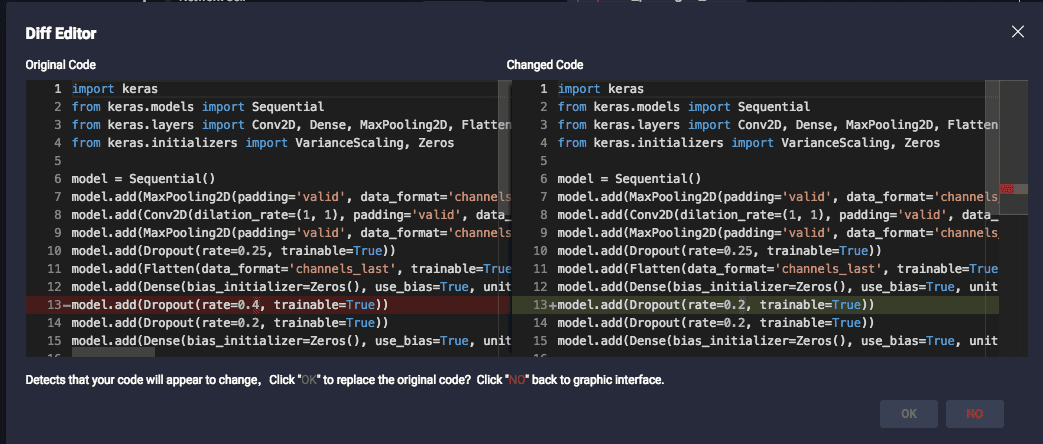This topic describes how to develop deep learning networks in a visualized manner.

## Background information

Neural networks were first introduced to the biology field to study the complicated structure of biological neurons. Multiple mathematical formulas were later used to simulate how the brain analyzes objects. This formed the basis for deep learning frameworks.

You can build deep learning networks through coding. However, a complex deep learning network contains tens or even hundreds of lines of code, and each network layer consists of multiple parameters, as shown in the following figure. After the number of network layers increases, it becomes more difficult to build a deep learning network through coding and the costs for maintaining the deep learning network also increase.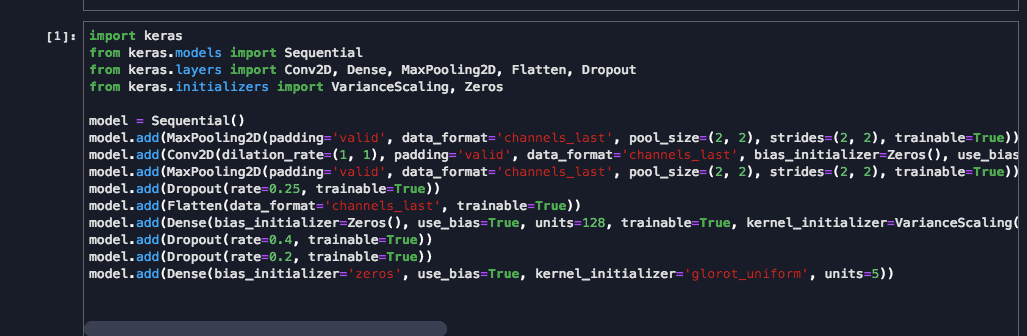The FastNeuralNetwork feature of Data Science Workshop (DSW) allows you to covert code for deep learning on graphs into a network architecture graph and supports graph editing. This improves model readability and makes it easier for you to maintain models.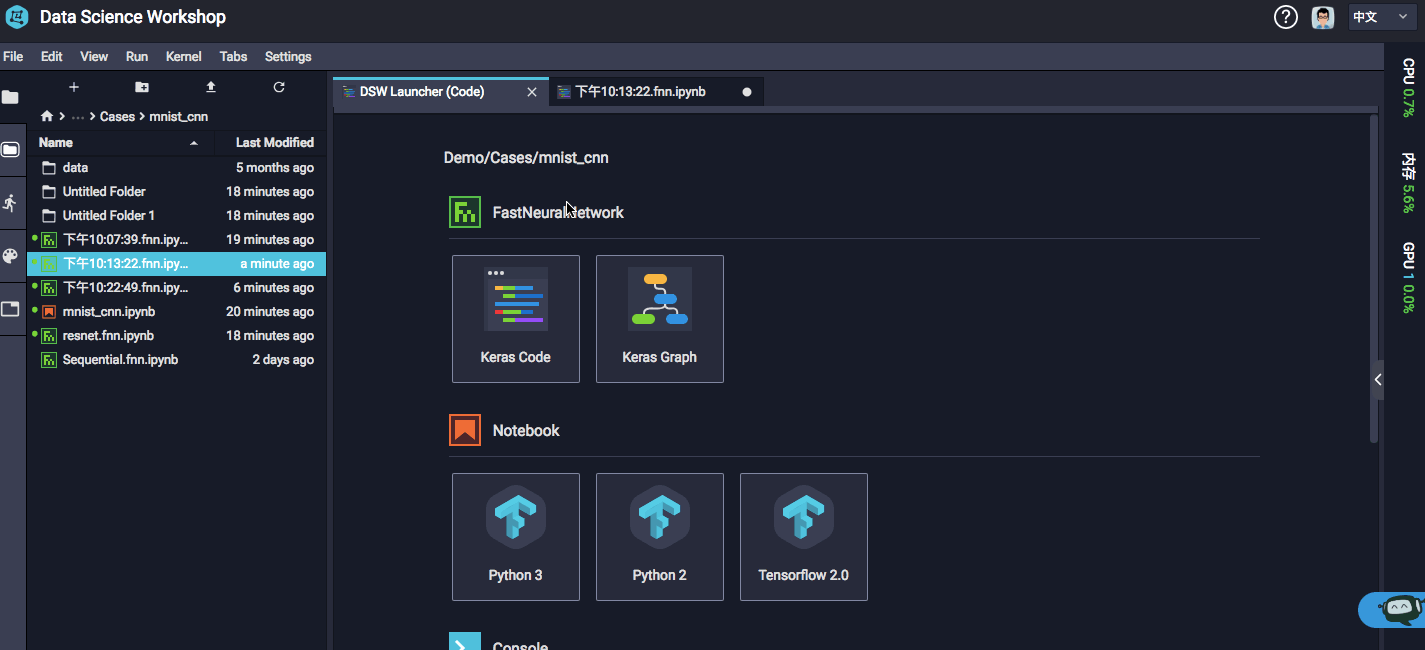## Use preset cases

If you are a first-time user of DSW, we recommend that you use preset cases to familiarize yourself with the procedure of how to develop deep learning networks in a visualized manner.

1. Download the preset case FNNDemo to build a deep learning network.
1. In the left-side navigation pane of the Data Science Workshop page, click the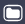icon.
2. Click the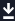icon next to FNNDemo.
2. Open the FNNDemo file.
1. In the left-side navigation pane, click the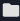icon.
2. Switch to the /Demo path.
You can switch to the path by using one of the following methods:
• Double-click Demo in the Name column.
• Right-click Demo in the Name column. In the shortcut menu that appears, click Open.
3. Switch to the /Demo/Cases path.
You can switch to the path by using one of the following methods:
• Double-click Cases in the Name column.
• Right-click Cases in the Name column. In the shortcut menu that appears, click Open.
4. Switch to the /demo/cases/FNNDemo path.
You can switch to the path by using one of the following methods:
• Double-click FNNDemo in the Name column.
• Right-click FNNDemo in the Name column. In the shortcut menu that appears, click Open.
You can open the file by using one of the following methods:
• Double-click the file in the Name column.
• Right-click the file in the Name column. In the shortcut menu that appears, click Open.
3. Edit the deep learning network by modifying the sample code. Then, save the changes. For more information, see Step 4 and Step 5 in the Custom network development section.

## Custom network development

The FastNeuralNetwork feature of DSW supports the following development modes:
• Keras Code: Write deep learning network code and convert the code into a graph.
• Keras Graph: Build a deep learning network on the canvas and convert the graph to code.
Keras Code is used as an example in this topic to describe how to develop deep learning networks in a visualized manner.
1. Click theicon to create a .ipynb file.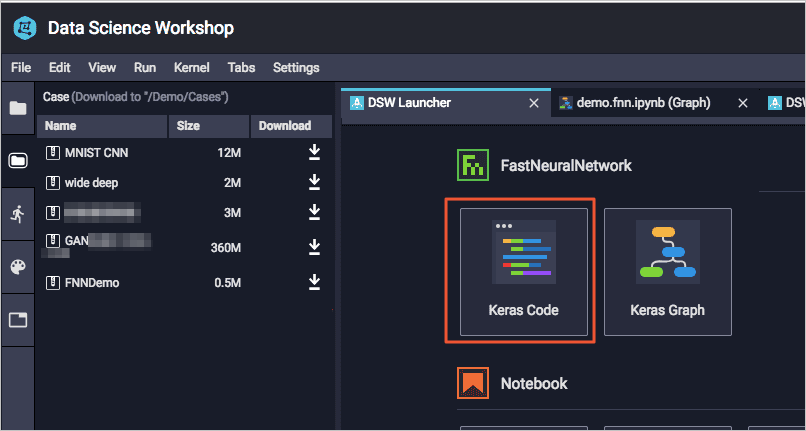2. On the interactive development page, build a deep learning network.
1. The following sample code is used to build the deep learning network:
``````import keras
from keras.models import Model
from keras.models import Sequential
from keras.layers import Conv2D, Dense, MaxPooling2D, Flatten, Dropout
from keras.initializers import VarianceScaling, Zeros
model = Sequential()
model.add(Conv2D(dilation_rate=(1, 1), padding='valid', data_format='channels_last', bias_initializer=Zeros(), use_bias=True, filters=64, strides=(1, 1), trainable=True, kernel_initializer=VarianceScaling(mode='fan_avg', seed=None, scale=1.0, distribution='uniform'), activation='relu', kernel_size=(3, 3)))
model.add(Dense(bias_initializer=Zeros(), use_bias=True, units=128, trainable=True, kernel_initializer=VarianceScaling(mode='fan_avg', seed=None, scale=1.0, distribution='uniform'), activation='relu'))
A Sequential model is created in this topic with `model` as the object.
``%show_model model``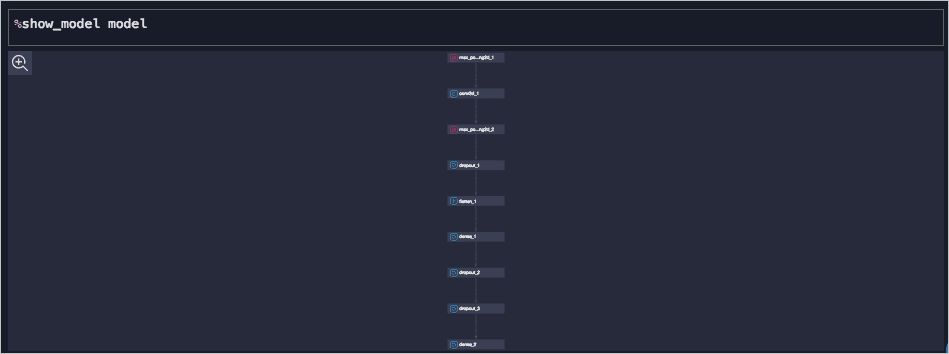3. Click the graph to open the graph editing page.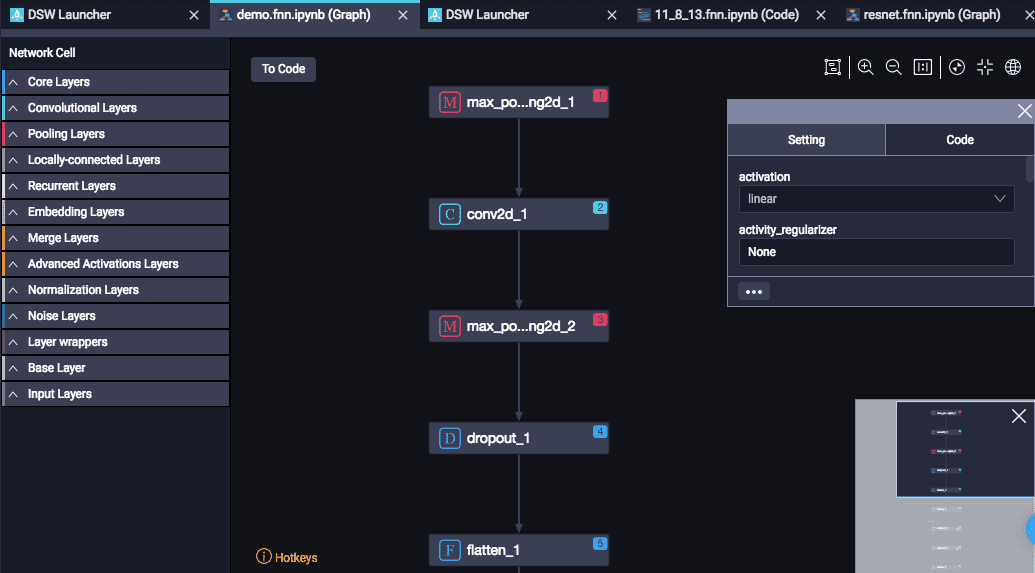Cells of the same function are connected, as shown in the following figure.The cells in the canvas are automatically mapped to the code, as shown in the following figure.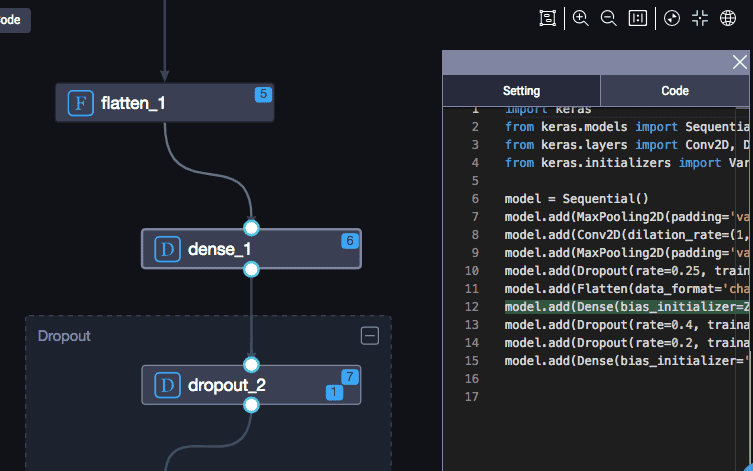To delete a cell or a connection in the canvas, select the code of the cell or connection, and press the Backspace key.
1. On the top of the canvas, click To Code. In the Diff Editor dialog box, the modification details are displayed.